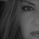# Indicators: Rainbow Charts Oscillator, Binary Wave and MAs

10539 views
10539
Rainbow Charts , by Mel Widner, is a trend detector. It uses recursively smoothed MAs (remember, this idea was proposed back in 1997 -- it was certainly cool back then!) and also builds an oscillator out of the MAs. Oscillator bands indicate the stability range.

I have also included a simple binary wave based on whether all the MAs are in an upward slope or not. If you see any value above 0.5 there, the trend is definitely up (all MAs pointing up).

Here's my complete list of indicators (With these 3, the total count should be above 100 now...will update the list later today)
```//
// @author LazyBear
//
// If you use this code in its original/modified form, do drop me a note.
//
study("Rainbow Charts Oscillator [LazyBear]", shorttitle="RCO_LB")
sma2=sma(close,2)
dsma2=sma(sma2,2)
tsma2=sma(dsma2,2)
qsma2=sma(tsma2,2)
psma2=sma(qsma2,2)
ssma2=sma(psma2,2)
s2sma2=sma(ssma2,2)
osma2=sma(s2sma2,2)
o2sma2=sma(osma2,2)
desma2=sma(o2sma2,2)

rmax=max(sma2,max(dsma2,max(tsma2,max(qsma2,max(psma2,max(ssma2,max(s2sma2,max(osma2,max(o2sma2,desma2)))))))))
rmin=min(sma2,min(dsma2,min(tsma2,min(qsma2,min(psma2,min(ssma2,min(s2sma2,min(osma2,min(o2sma2,desma2)))))))))
rosc=100*(close-((sma2+dsma2+tsma2+qsma2+psma2+ssma2+s2sma2+osma2+o2sma2+desma2)/10))/(highest(close,10)-lowest(close,10))
rbl=-100*(rmax-rmin)/(highest(close,10)-lowest(close,10))
rbu=-rbl //100*(rmax-rmin)/(highest(close,10)-lowest(close,10))
ml=plot(0)
ll=plot(rbl, color=gray)
ul=plot(rbu, color=gray)
plot(rosc, color=rosc>=0?green:red, linewidth=3, style=histogram)

fill(ll, ml, red)
fill(ml, ul, green)```Just noticed that Rahul Mohindar Oscillator (https://www.tradingview.com/v/efHJsedw/) builds directly on top of Rainbow Charts Oscillator. RMO is nothing but an 81EMA of RCO!

Rahul added some more 2nd/3rd EMA derivatives to generate buy/sell signals, but the core logic is Mel Widner's. Wonder if there was a deal worked out or is just a plain rip-off!